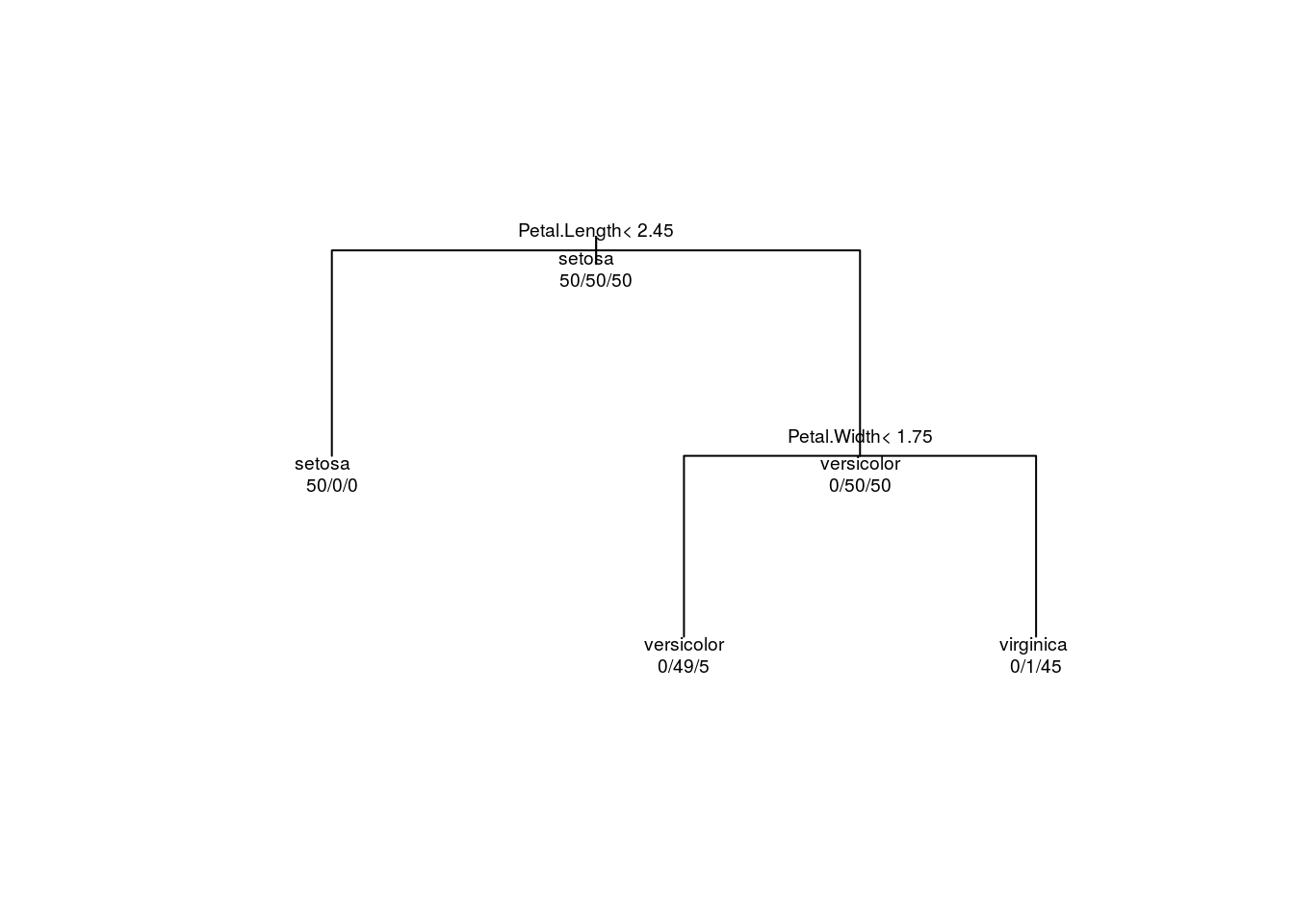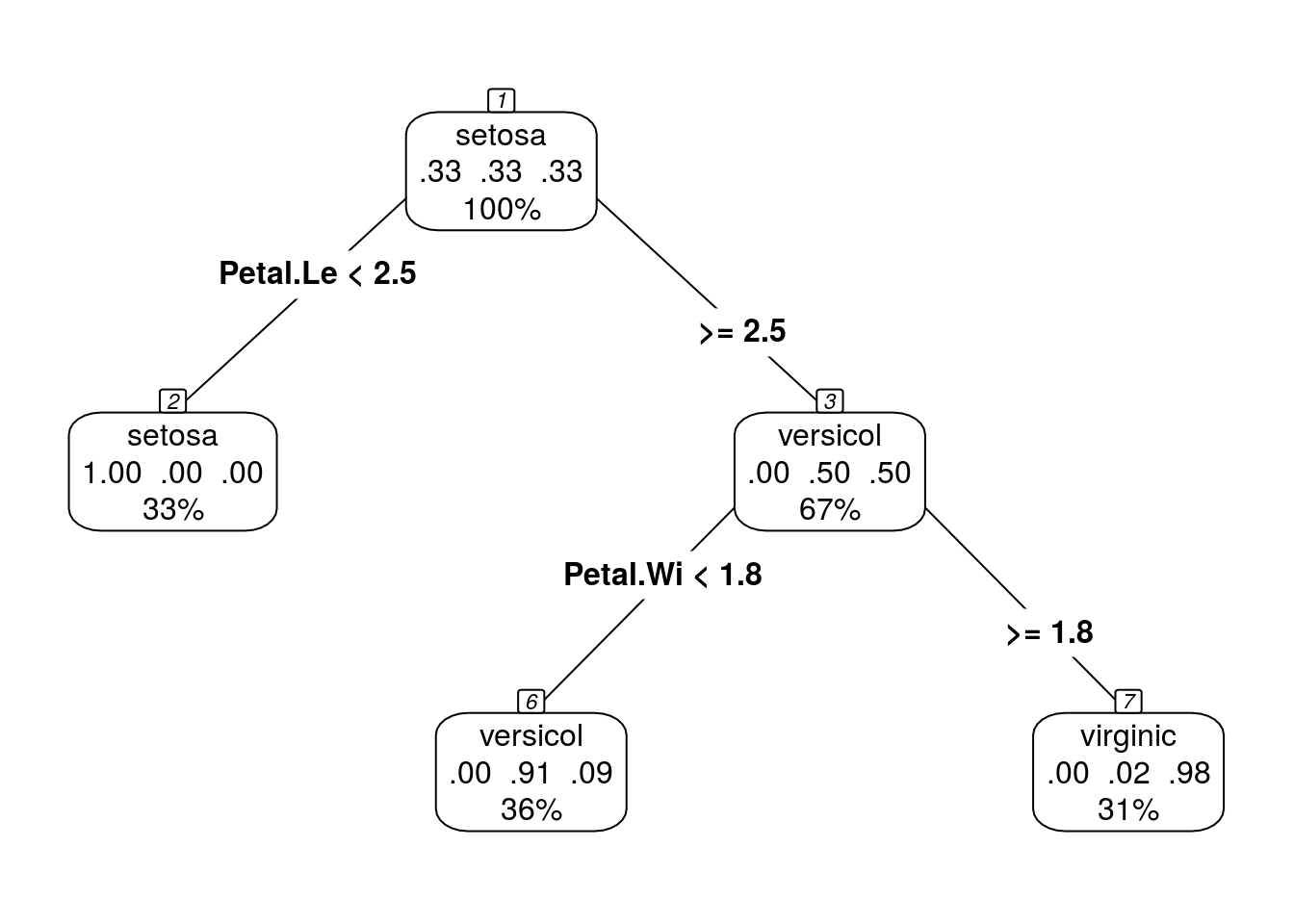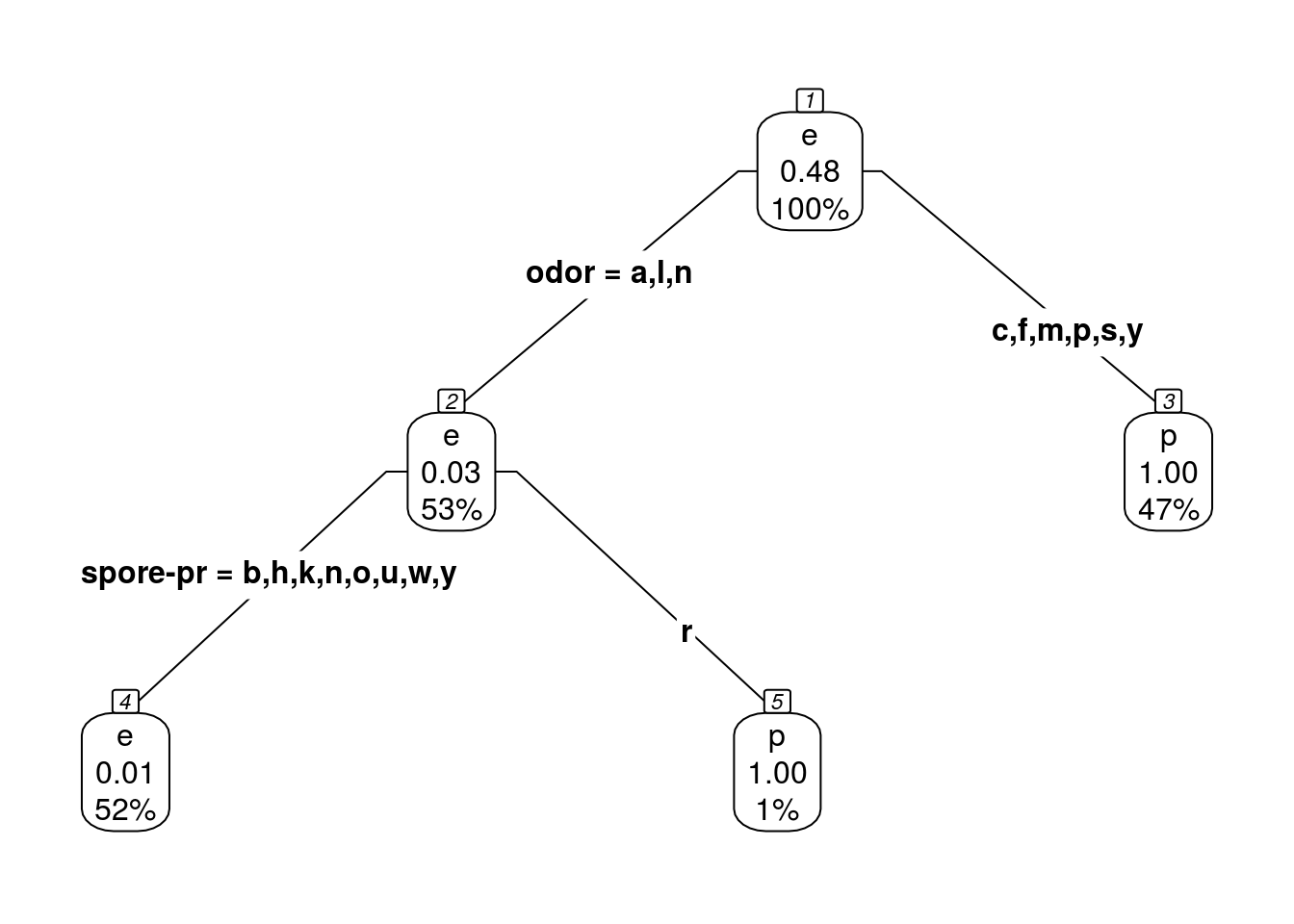For theoretical explanations see course slides, and http://www.rdatamining.com/docs/r-and-data-mining-examples-and-case-studies chapter 4

# Information and Classification

## Entrophy

Compute entrophy

require(DescTools)
## Loading required package: DescTools
x <- as.factor(c("a","b","a","a","b","b"))
y <- as.factor(c("a","b","a","a","a","a"))
z <- as.factor(c("a","a","a","a","a","a"))
Entropy(table(x))
##  1
Entropy(table(y))
##  0.6500224
Entropy(table(z))
##  0

EXERCISE

1. Compute the enthrophy of wine types in wine data set from rattle.data.
2. Compute the enthrophy of wine types for a subset of data that has alcohol level above the average.

## Exploring information value of variables for classification: info gain

Classification trees can get very complicated and as a result fails to explain the phenomenon. So one must try to include as few variables as possible, by looking at the information gain:

data(iris)
library(FSelector)
weights <- information.gain(Species~., iris)
print(weights)
##              attr_importance
## Sepal.Length       0.4521286
## Sepal.Width        0.2672750
## Petal.Length       0.9402853
## Petal.Width        0.9554360

EXERCISE 1. Compute the importance of attributes in wine dataset. 2. Compute the importance of attributes in iris dataset.

## Tree based classification

require(rpart)
## Loading required package: rpart
data(iris)
tree <- rpart(Species ~ ., data=iris, method="class")
summary(tree)
## Call:
## rpart(formula = Species ~ ., data = iris, method = "class")
##   n= 150
##
##     CP nsplit rel error xerror       xstd
## 1 0.50      0      1.00   1.19 0.04959167
## 2 0.44      1      0.50   0.68 0.06096994
## 3 0.01      2      0.06   0.11 0.03192700
##
## Variable importance
##  Petal.Width Petal.Length Sepal.Length  Sepal.Width
##           34           31           21           14
##
## Node number 1: 150 observations,    complexity param=0.5
##   predicted class=setosa      expected loss=0.6666667  P(node) =1
##     class counts:    50    50    50
##    probabilities: 0.333 0.333 0.333
##   left son=2 (50 obs) right son=3 (100 obs)
##   Primary splits:
##       Petal.Length < 2.45 to the left,  improve=50.00000, (0 missing)
##       Petal.Width  < 0.8  to the left,  improve=50.00000, (0 missing)
##       Sepal.Length < 5.45 to the left,  improve=34.16405, (0 missing)
##       Sepal.Width  < 3.35 to the right, improve=19.03851, (0 missing)
##   Surrogate splits:
##       Petal.Width  < 0.8  to the left,  agree=1.000, adj=1.00, (0 split)
##       Sepal.Length < 5.45 to the left,  agree=0.920, adj=0.76, (0 split)
##       Sepal.Width  < 3.35 to the right, agree=0.833, adj=0.50, (0 split)
##
## Node number 2: 50 observations
##   predicted class=setosa      expected loss=0  P(node) =0.3333333
##     class counts:    50     0     0
##    probabilities: 1.000 0.000 0.000
##
## Node number 3: 100 observations,    complexity param=0.44
##   predicted class=versicolor  expected loss=0.5  P(node) =0.6666667
##     class counts:     0    50    50
##    probabilities: 0.000 0.500 0.500
##   left son=6 (54 obs) right son=7 (46 obs)
##   Primary splits:
##       Petal.Width  < 1.75 to the left,  improve=38.969400, (0 missing)
##       Petal.Length < 4.75 to the left,  improve=37.353540, (0 missing)
##       Sepal.Length < 6.15 to the left,  improve=10.686870, (0 missing)
##       Sepal.Width  < 2.45 to the left,  improve= 3.555556, (0 missing)
##   Surrogate splits:
##       Petal.Length < 4.75 to the left,  agree=0.91, adj=0.804, (0 split)
##       Sepal.Length < 6.15 to the left,  agree=0.73, adj=0.413, (0 split)
##       Sepal.Width  < 2.95 to the left,  agree=0.67, adj=0.283, (0 split)
##
## Node number 6: 54 observations
##   predicted class=versicolor  expected loss=0.09259259  P(node) =0.36
##     class counts:     0    49     5
##    probabilities: 0.000 0.907 0.093
##
## Node number 7: 46 observations
##   predicted class=virginica   expected loss=0.02173913  P(node) =0.3066667
##     class counts:     0     1    45
##    probabilities: 0.000 0.022 0.978
plot(tree,margin=0.2)
text(tree, use.n=TRUE, all=TRUE, cex=.6)#alternative visualization
require(rpart.plot)
## Loading required package: rpart.plot
prp(tree,type=4,extra="auto",nn=TRUE)## Confusion matrix for classification

require(rpart)
data(iris)
tree <- rpart(Species ~ ., data=iris, method="class")
require(caret)
## Loading required package: caret
## Loading required package: lattice
## Loading required package: ggplot2
##
## Attaching package: 'caret'
## The following objects are masked from 'package:DescTools':
##
##     MAE, RMSE
pred <- predict(tree, newdata=iris,type="class")
confusionMatrix(pred, iris$Species) ## Confusion Matrix and Statistics ## ## Reference ## Prediction setosa versicolor virginica ## setosa 50 0 0 ## versicolor 0 49 5 ## virginica 0 1 45 ## ## Overall Statistics ## ## Accuracy : 0.96 ## 95% CI : (0.915, 0.9852) ## No Information Rate : 0.3333 ## P-Value [Acc > NIR] : < 2.2e-16 ## ## Kappa : 0.94 ## Mcnemar's Test P-Value : NA ## ## Statistics by Class: ## ## Class: setosa Class: versicolor Class: virginica ## Sensitivity 1.0000 0.9800 0.9000 ## Specificity 1.0000 0.9500 0.9900 ## Pos Pred Value 1.0000 0.9074 0.9783 ## Neg Pred Value 1.0000 0.9896 0.9519 ## Prevalence 0.3333 0.3333 0.3333 ## Detection Rate 0.3333 0.3267 0.3000 ## Detection Prevalence 0.3333 0.3600 0.3067 ## Balanced Accuracy 1.0000 0.9650 0.9450 ## Tree based classification with Rattle Use Rattle -> Model -> Tree. Then “Evaluate” to see confusion matrix. Examine the command log and note similarities to above commands You may also try random forest models in Rattle ## Exercise and review questions Download and imort the Mushroom data set from Kaggle and save in variable “mushrooms”: https://www.kaggle.com/uciml/mushroom-classification/data library(readr) mushrooms <- read_csv("~/Downloads/mushrooms.csv") ## Parsed with column specification: ## cols( ## .default = col_character() ## ) ## See spec(...) for full column specifications. 1. Consider the edible/poisonous mix in the data set marked as “e” and “p” respectively: summary(mushrooms$class)
##    Length     Class      Mode
##      8124 character character

Write the mathematical expression to compute the entropy of this mix. Then use the Entropy function to actually find its value.

1. Consider the information gains:
data(iris)
library(FSelector)
weights <- information.gain(class ~ ., data=mushrooms)
print(weights)
##                          attr_importance
## cap-shape                    0.033823296
## cap-surface                  0.019817239
## cap-color                    0.024987459
## bruises                      0.133347298
## odor                         0.628043316
## gill-attachment              0.009818449
## gill-spacing                 0.069926895
## gill-size                    0.159530856
## gill-color                   0.289026795
## stalk-shape                  0.005210230
## stalk-root                   0.093448465
## stalk-surface-above-ring     0.197356746
## stalk-surface-below-ring     0.188462888
## stalk-color-above-ring       0.175952066
## stalk-color-below-ring       0.167336519
## veil-type                    0.000000000
## veil-color                   0.016508698
## ring-number                  0.026653359
## ring-type                    0.220435714
## spore-print-color            0.333199258
## population                   0.139986632
## habitat                      0.108708771

Which variable you think should be at the root of the classification tree?

1. Consider the classification tree below
require(rpart)
tree <- rpart(class ~ ., data=mushrooms, method="class")
require(rpart.plot)
prp(tree,type=4,extra="auto",nn=T)(a) How would you describe node 5 in the classification tree in plain English? (b) What percentage of the initial sample is in node 5? (c) What is the probability that an item in node 2 is poisonous?

1. Consider the confusion matrix below:
require(caret)
pred <- predict(tree, newdata=mushrooms,type="class")
cm<- confusionMatrix(pred, mushrooms$class) cm$table
##           Reference
## Prediction    e    p
##          e 4208   48
##          p    0 3868
1. How many of the poisonous items are classified as edible?
2. How many of the edible items are classified as poisonous?
3. What percentage of items in total are wrongly classified (i.e. the error rate of the classifier)?

# Further exercises and case studies

## Exercise

Get the wine data https://archive.ics.uci.edu/ml/datasets/Wine+Quality Find a tree model for wine quality being more than 5.

## Tutorial Case study: Credit card cutomers

Get the data from https://archive.ics.uci.edu/ml/datasets/default+of+credit+card+clients You are recommended to (1)open data from Excel, correct column names NOT to include spaces, (2) export as csv then import to Rstudio or Rattle

Follow this tutorial http://www.askanalytics.in/2015/10/decision-tree-in-r-telecom-case-study.html but use the above data

## Exercise: Churn analysis

You can obtain the data for this case at https://www.ibm.com/communities/analytics/watson-analytics-blog/predictive-insights-in-the-telco-customer-churn-data-set/

1. Find the information gain of variables.
2. Build a decision tree of the churn output, and vew the error matrix.
3. What are the alternatives for modeling?